# Reversing Deterministic Finite Automata

Prerequisite – Designing finite automata
Reversal: We define the reversed languageto be the language, whereSteps to Reversal:

1. Draw the states as it is.
2. Add a new single accepting state
3. Make the accepting state the initial state and initial state the accepting state
4. Invert the transition function into a transition relation
5. Remove any states that are no longer necessary

Note :

Reversing deterministic finite automaton results in a non-deterministic finite automaton. This can then be converted to a deterministic finite automaton by the conversion algorithm.

The reversal process goes like this: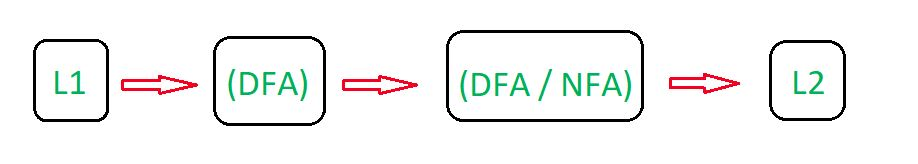Let’s understand the Reversal process in Deterministic Finite Automata (DFA) with the help of the example below.

Example 1:

We propose a deterministic finite automaton for the language of words beginning with the symbol ‘a’:We can construct the following state transition diagram: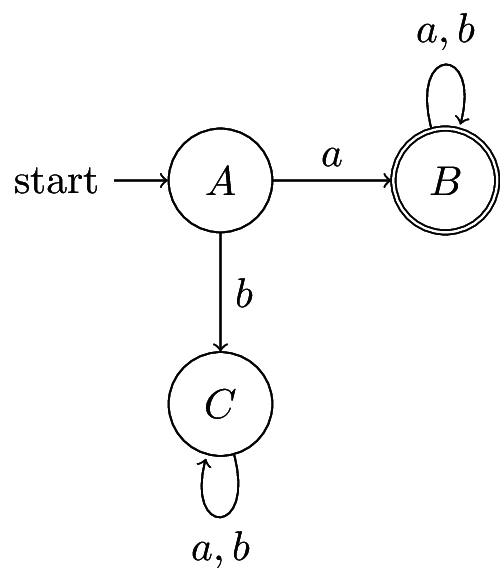We now want to construct the finite automaton that will accept the reverse of this language,.

First, we add a new single accepting state. This is because eventually we will need to swap the accepting and initial states, and there can only be a single initial state.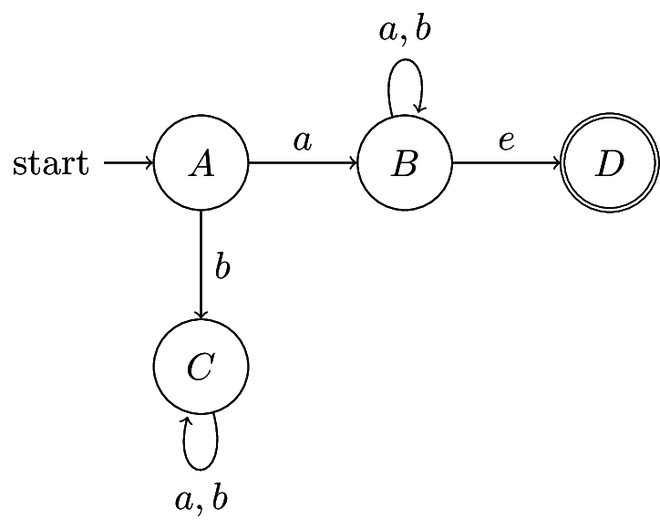Then, switch the initial and accepting states: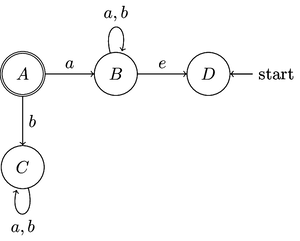Invert the transition function into a transition relation: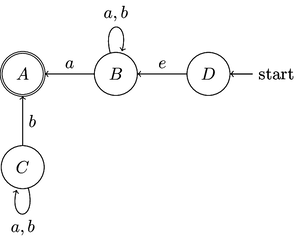Whilst this is a valid solution, the node marked as C is dangling since it has no incident edges. Therefore it can be removed to give a clean, final result: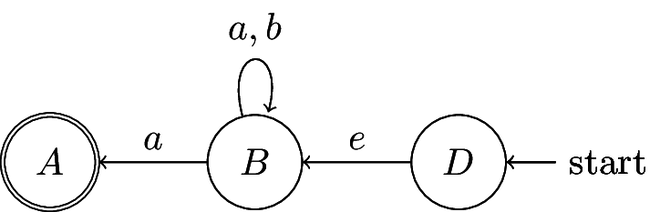This resulting finite automaton is a non-deterministic finite automaton. Therefore the resulting reversed language is regular.

Explanation:

1. Same states (A, B, C) are drawn as present in an original state diagram
2. Since A is the initial state make it the final state.
3. Since B is the final state make it the initial state.
4. Reversing the edges, since previously edges point A to B and A to C . Change the direction, now edges points toward B to A and C to A.
5. Assign the values as same as the original.
6. Draw the loop as in the original state diagram
7. Since there is no incident edge on state C so, we can reduce this state C.
8. Since there is no transition for ‘a’ and ‘b’ in state A . Therefore resultant FA is NFA.

Example-2:
Designing a DFA for the set of strings over {a, b} such that string of the language contains an even number of ‘a’. The desired language will be formed:

L1 = {, aa, aab, aba, aab, aababa, .......}

In L1, each string has an even number of elements a.

State Transition Diagram for the language L1: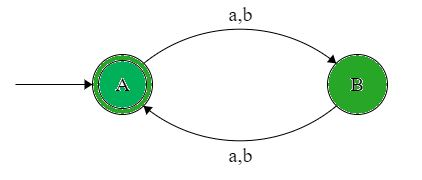This DFA accepts all the strings containing an even number of elements a. Here, State A is the initial state, and state A is the final state. Now, Reversing the L1 language which gives the final result of the language L2.

State Transition Diagram of L2 (reverse of L1):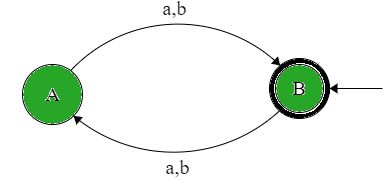Thus as we see that L2 has been obtained through the reversal process and this final DFA accepts all the strings containing an even number of element a which is the same as the original language.

L2 = {, aa, aab, aba, aab, aababa, .......}

Explanation:

1. Same states (A, B) are drawn as present in an original state diagram.
2. Since A is the initial state make it the final state.
3. Since A is the final state make it the initial state.
4. Reversing the edges, since previously edges point A to B and so on. Change the direction, now edges points toward B to A and so on.
5. Assign the values as same as the original.
6. Since there is a transition for ‘a’ and ‘b’ in states A and B.Therefore resultant FA is DFA.
7. Since the State diagram after and before the reversal is the same. Therefore L1 = L2